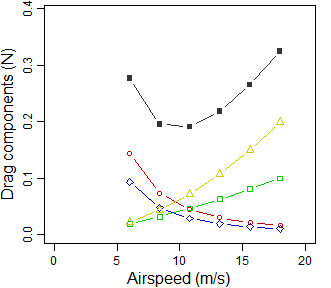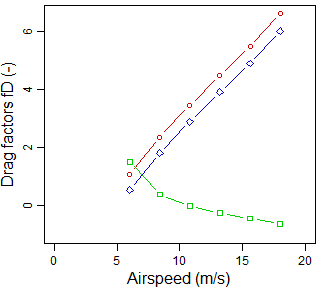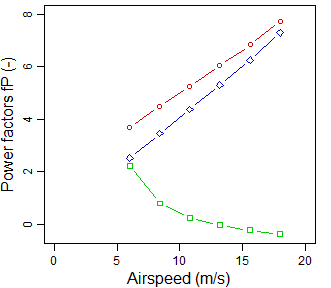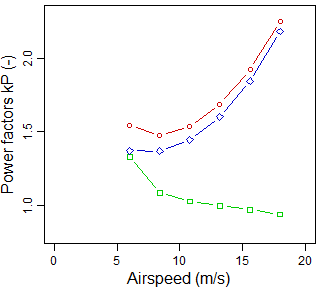# AFPT – Aerodynamic model

#### 2020-03-18

library('afpt')

This document describes the aerodynamic model that this package uses for estimating flight performance of flapping flight (see also Klein Heerenbrink et al., 2015). The model first calculates the drag of the bird when it is not flapping its wings (i.e. it is not producing thrust). Then factors are computed that adjust the non-flapping drag for the effect of flapping the wings at a specified frequency (and strokeplane angle).

The process described here is performed by the function computeFlappingPower(). We first define a bird:

myBird <- Bird(
massTotal = 0.215,
wingSpan = 0.67,
wingArea = 0.0652,
name = 'Jackdaw',
name.scientific = 'Corvus monedula',
type = 'passerine',
source = 'KleinHeerenbrink M, Warfvinge K and Hedenström A 2016 J.Exp.Biol. 219: 10, 1572--1581'
)

Compute powercurve for a range of airspeeds:

speed <- seq(6,18,length.out=6) #  airspeed in m/s
powercurve <- computeFlappingPower(myBird,speed)

## Non-flapping drag components

The aerodynamic model assumes 4 distinct sources of drag:

• Induced drag: $D^\prime_\mathrm{ind} = \frac{L^2}{q \pi b^2} ,$ where $$q = \frac{1}{2} \rho U^2$$.

• Zero lift profile drag: $D^\prime_\mathrm{pro,0} = q C_{D_\mathrm{pro,0}} S,$

• Lift dependent profile drag: $D^\prime_\mathrm{pro,2} = k_\mathrm{p}\frac{L^2}{q S},$

• Parasite drag: any other forces that act on the wings as external sources of drag, e.g.:

• body drag $D^\prime_\mathrm{body} = q C_{D_\mathrm{b}} S_\mathrm{b},$
• drag from feet, carried objects, etc.,
• apparent drag due to climbing $D^\prime_\mathrm{climb} = W \sin(\gamma) ,$ with $$\gamma$$ the climb angle.

Some of the variables used here are relatively easy to measure on a bird in a standardized way (see Pennycuick, 2008):

• $$m$$ body mass (in steady flight $$L = W = m g$$, with $$g$$ the gravitational acceleration)
• $$b$$ wing span
• $$S$$ wing area

which we used to construct myBird.

The coefficients $$C_{D_\mathrm{pro,0}}$$, $$k_\mathrm{p}$$ and $$C_{D_\mathrm{b}}$$ are more difficult to determine, and the literature on this subject offers a wide range of possibilities. For these coefficients, the Bird() constructor assumes default values, which were used for the computation of the powercurve above. The default settings return in the following non-flapping drag components:

par(mar=c(3.1,3.1,0.4,1.1),mgp=c(1.9,0.7,0.0),cex=0.75)
with(powercurve , plot( speed, Dnf.ind+Dnf.pro0+Dnf.pro2+Dnf.par,
type='b', pch=15, col='grey20',
xlab=NA, ylab=NA, xlim=c(0,20), ylim=c(0,0.39)))
with(powercurve , lines( speed, Dnf.ind, type='b', pch=21, col='red3'))
with(powercurve , lines( speed, Dnf.pro0, type='b', pch=22, col='green3'))
with(powercurve , lines( speed, Dnf.pro2, type='b', pch=23, col='blue3'))
with(powercurve , lines( speed, Dnf.par, type='b', pch=24, col='yellow3'))
mtext(side = 1, line = 2,'Airspeed (m/s)')
mtext(side = 2, line = 2,'Drag components (N)')Drag components – Black: total drag; red circles: induced drag; green squares: zero-lift profile drag; blue diamonds: lift-dep. profile drag; yellow triangles: parasitic drag.

### Zero lift profile drag

In this model we assume the zero lift profile drag is caused by the friction of a laminar boundary layer over the aerofoil. Specifically the model uses $C_{D_\mathrm{pro,0}} = \frac{2.66}{\sqrt{Re_c}},$ where $$Re_c$$ is the Reynolds number for the mean chord length $$c$$ at air speed $$U$$ with kinematic viscosity $$\nu$$: $Re_c = \frac{U c}{\nu}.$ This is the solution for a flat plate laminar boundary layer in a paralel flow. At large Reynolds numbers, the laminar boundary layer may transition to a turbulent boundary layer somewhere along the aerofoil (Anderson, 2007), however, this typically occurs at higher Reynolds numbers than those experienced by birds.

### Lift dependent drag coefficient

The coefficient for lift-dependent profile drag, $$k_\mathrm{p}$$ indicates how efficient the aerofoil cross-section (note: not the wing) is at producing lift. For an aerofoil at low Reynolds number, $$k_\mathrm{p}$$ can be relatively high (Spedding and McArthur (2010)). At higher Reynolds numbers (i.e. large bird and/or high flight speed) this factor tends to decrease. Additionally, birds with very high wing loading also usually have highly cambered aerofoils. This has the effect that the minimum profile drag for the aerofoil is shifted towards a higher lift coefficient. If a bird has only a narrow range of speeds at which it flies, there is good reason for the aerofoil to be optimized for this specific condition. In that case a value $$k_\mathrm{p} = 0$$ may be more appropriate.

The Bird() constructor will by default assume $$k_\mathrm{p}=0.03$$. Custom values can be set by providing the numeric argument coef.profileDragLiftFactor to the function (or by reassigning this property in the bird object).

### Body drag coefficient

The body drag coefficient has turned out to be a difficult parameter to obtain for birds. Early wind tunnel experiments with frozen bird bodies, resulted in values between 0.2 and 0.4, e.g. Pennycuick et al. (1988). Tucker (1990) showed these wind tunnel measurements suffered from interference drag of the mounting strut. An even broader range of values was obtained from radar trackings of diving passerines by Hedenström and Liechti (2001). Additionally, the applicability of this paramter also depends on the used reference area $$S_\mathrm{b}$$. Pennycuick et al. (1988) found the relationship $$S_\mathrm{b} = 0.00813 m^{0.666}$$ with body mass for waterfowl and raptors, whereas Hedenström and Rosén (2003) found a relationship $$S_\mathrm{b} = 0.0129 m^{0.614}$$ for passerines.

The default value for body drag coefficient is $$C_{D_\mathrm{b}} = 0.2$$. This seems to be a recurring value in experiments, e.g. Henningsson and Hedenström (2011) and KleinHeerenbrink et al. (2016). The default body frontal area is determined based on the body mass relationship of Hedenström and Rosén (2003) in case of type="passerine" or the body mass relationship of Pennycuick et al. (1988) for type="other".

Both body frontal area and the body drag coefficient can be customized, either by specification in the Bird() constructor, or by reassignment in the bird object. Here it should be kept in mind that the body drag coefficient needs to match the body frontal area.

with(myBird,coef.bodyDragCoefficient*bodyFrontalArea)
##  0.001004007

In some literature, values for the product $$C_{D_\mathrm{b}}S_\mathrm{b}$$ can be found. This convention can be accomodated as:

myBird$coef.bodyDragCoefficient <- 0.001004007 # the product CDb*Sb myBird$bodyFrontalArea <- 1 #  unit area
with(myBird,coef.bodyDragCoefficient*bodyFrontalArea)
##  0.001004007

Alternatively, some literature provides body drag coefficients relative to the wing reference area. This convention has the advantage that all drag coefficients can be added as a measure of total drag. This convention can be accomodated as:

myBird$coef.bodyDragCoefficient <- 0.01539888 # CDb relative to wing reference area myBird$bodyFrontalArea <- myBird$wingArea # unit area with(myBird,coef.bodyDragCoefficient*bodyFrontalArea) ##  0.001004007 ## Flapping model ### Wingbeat frequency, strokeplane angle and amplitude In the numerical computations on which this model is based (Klein Heerenbrink et al., 2015), the wingbeat amplitude was optimized to produce minimum induced power for a given wingbeat frequency and strokeplane angle. This means that the wingbeat amplitude is an output, not an input, of the model. The strokeplane angle is by default optimized by computeFlappingPower(), but it can also be provided as an input. Wingbeat frequency is purely an input to the flight model. Generally, a optimum wingbeat frequency for which aerodynamic power is minimum, does not exist within the typical range of wingbeat frequencies used by the bird. All three wingbeat parameters are returned by the computeFlappingPower() function. For this example, the strokeplane angle has been optimized to minimize aerodynamic power. Frequency is the default reference frequency of the bird. powercurve[c('speed','amplitude','strokeplane','frequency')] ## speed amplitude strokeplane frequency ## 1 6.0 38.19161 39.30489438 5.948083 ## 2 8.4 34.39946 26.56562747 5.948083 ## 3 10.8 36.32754 18.76822057 5.948083 ## 4 13.2 41.27462 13.54717870 5.948083 ## 5 15.6 47.55337 9.12191443 5.948083 ## 6 18.0 53.82718 0.06608206 5.948083 ### Upstroke wing flexion Most vertebrates flex their wings during the upstroke. In the model, the degree of flexion is linked to the wingbeat amplitude $$\theta$$: $\frac{b_\mathrm{u.s.}}{b} = \cos (\theta).$ This formulation ensures that there is no wing flexion when the bird is not flapping its wings. For small wingbeat amplitudes, it results in that the wing tips are raised following a nearly straight trajectory. Alternative upstroke flexion models are to be investigated in future work. ### Thrust requirement The drag components in flapping flight can be obtained by multiplying the non-flapping components with a factor that depends on the wingbeat kinematics: $D_i = k_{D_i} D^\prime_i,$ using $$i$$ for each drag component. The factors $$k_{D_i}$$ scale with the thrust requirement $${T}/{L}$$, the ratio between thrust and lift: $k_{D_i} = 1 + f_{D_i}(k_f,\phi) \frac{T}{L},$ where $$f_{D_i}(k_f,\phi)$$ is a specific function for each of the drag components, depending on strokeplane angle $$\phi$$ and the reduced frequency $$k_f = 2\pi f b / U$$ ( $$f$$ being the wingbeat frequency). The parasitic drag terms are assumed to be independent of wingbeat, i.e. $$f_{D_\mathrm{par}}=0$$. The values of $$f_{D_\mathrm{ind}}$$, $$f_{D_\mathrm{pro,0}}$$ and $$f_{D_\mathrm{pro,2}}$$, are computed using fD.ind(kf,phi), fD.pro0(kf,phi) and fD.pro2(kf,phi): par(mar=c(3.1,3.1,0.4,1.1),mgp=c(1.9,0.7,0.0),cex=0.75) kf <- 2*pi*myBird$wingSpan*myBird$wingbeatFrequency / speed # reduced frequency phi <- powercurve$strokeplane*pi/180 #  strokeplane angle in radians (optimized)
fD <- data.frame(
ind = fD.ind(kf,phi), #  induced drag
pro0 = fD.pro0(kf,phi), #  zero lift profile drag
pro2 = fD.pro2(kf,phi), #  lift dep. profile drag
par = 0 # parasitic drag is wingbeat independent
)
plot( speed, fD$ind, type='b', pch=21, col='red3', xlab=NA, ylab=NA, xlim=c(0,20), ylim=c(-1,6.6)) lines( speed, fD$pro0, type='b', pch=22, col='green3')
lines( speed, fD$pro2, type='b', pch=23, col='blue3') mtext(side = 1, line = 2,'Airspeed (m/s)') mtext(side = 2, line = 2,'Drag factors fD (-)')Note how the factors for the lift dependent components increase with speed, whereas the $$f_{D_\mathrm{pro,0}}$$ decreases and even takes negative values. The thrust required for steady flight, needs to balance the total drag in flapping flight: $T = {\sum k_{D_i} D^\prime_i}$ This means the thrust itself depends on the thrust requirement: $T = {\sum D^\prime_i} + {\sum f_{D,i} D^\prime_i}\frac{T}{L} ,$ but because of the linear form of $$k_D$$, this equation is easily solved: $\frac{T}{L} = \frac{\sum D^\prime_i}{L - \sum f_{D,i} D^\prime_i}$ thrustratio <- apply(powercurve[,grep('Dnf',names(powercurve))],1,sum) / (powercurve$L - apply(fD*powercurve[,grep('Dnf',names(powercurve))],1,sum))

With the thrust ratio known, we could explicitly compute the drag factors $$k_{D_i}$$, but unless we are going to measure the drag components empirically, this is not very usefull. However, as described in the next section, the power components also depend on this thrust ratio.

### Aerodynamic power of flapping flight

Mechanical power is the rate of energy related with exerting a force onto a moving object; mathematically $$P=\mathbf{F}\cdot\mathbf{V}$$. Aerodynamic power of flight can therefore be expressed as the product of thrust and airspeed $$P = T U$$. However, because the bird is flapping its wings, producing variable aerodynamic forces throughout the wingbeat, the aerodynamic power for flapping flight needs to be modified. The required correction is very similar to that used for the drag in flapping flight: $P = \sum k_{P_i} D^\prime_i U,$ where $k_{P_i} = 1 = f_{P_i} (k_f,\phi) \frac{T}{L}.$ We again assume the parasitic drag components are independent of the wingbeat: $$f_{P_\mathrm{par}}=0$$. The values of $$f_{P_\mathrm{ind}}$$, $$f_{P_\mathrm{pro,0}}$$ and $$f_{P_\mathrm{pro,2}}$$, are computed using fP.ind(kf,phi), fP.pro0(kf,phi) and fP.pro2(kf,phi):

fP <- data.frame(
ind = fP.ind(kf,phi), #  induced power
pro0 = fP.pro0(kf,phi), #  zero lift profile power
pro2 = fP.pro2(kf,phi), #  lift dep. profile power
par = 0 #  parasitic power is wingbeat independent
)

As can be seen below, the general behaviour of $$f_{P_i}$$ is rather similar to $$f_{D_i}$$, except for that the lift dependent factors are significantly raised. Combined with the required thrust ratio, we can also look at the power factors $$k_{P_i}$$:

kP <- 1 + fP*thrustratio

Note that the factors $$k_{D_i}$$ and $$k_{P_i}$$ are also returned in the output of computeFlappingPower().

par(mar=c(3.1,3.1,0.4,1.1),mgp=c(1.9,0.7,0.0),cex=0.75)
plot( speed, fP$ind, type='b', pch=21, col='red3', xlab=NA, ylab=NA, xlim=c(0,20), ylim=c(-0.4,8)) lines( speed, fP$pro0, type='b', pch=22, col='green3')
lines( speed, fP$pro2, type='b', pch=23, col='blue3') mtext(side = 1, line = 2,'Airspeed (m/s)') mtext(side = 2, line = 2,'Power factors fP (-)') plot( speed, kP$ind, type='b', pch=21, col='red3',
xlab=NA, ylab=NA, xlim=c(0,20), ylim=c(0.8,2.3))
lines( speed, kP$pro0, type='b', pch=22, col='green3') lines( speed, kP$pro2, type='b', pch=23, col='blue3')
mtext(side = 1, line = 2,'Airspeed (m/s)')
mtext(side = 2, line = 2,'Power factors kP (-)')## Model limits

The functions $$f_{D_i}$$ and $$f_{P_i}$$ are somewhat arbitrary polynomial functions that were fitted to a set of numerically obtained data. These data points were computed for a range of thrust ratios $$0\leq T/L \leq 0.3$$, reduced frequencies $$1 \leq k_f \leq 6$$ and strokeplane angles $$0 \leq \phi \leq 50^\circ$$. The functions are a good representation within these limits, but as the fitting functions do not have a physical basis, they should not be used for extrapolations outside these limits. For this reason, computeFlappingPower() returns several flags:

powercurve[,grep('flags',names(powercurve))]
##   flags.redFreqLo flags.redFreqHi flags.thrustHi flags.speedLo
## 1           FALSE           FALSE          FALSE         FALSE
## 2           FALSE           FALSE          FALSE         FALSE
## 3           FALSE           FALSE          FALSE         FALSE
## 4           FALSE           FALSE          FALSE         FALSE
## 5           FALSE           FALSE          FALSE         FALSE
## 6           FALSE           FALSE          FALSE         FALSE

These indicate low reduced frequency, high reduced frequency, high thrust requirement and low speed, respectively. The first four relate to the range of the numerical data set. The limits for strokeplane angle are not flagged, as these are either provided as input by the user, or limited by the optimization algorithm. The last flag, low speed, relates to the validity of the non-flapping drag model. Below this speed the downwash required to produce lift becomes large compared to the forward flight speed, so that it needs to be taken into account as the airspeed experienced by the bird (note that the expression for induced drag goes to infinity at zero flight speed).